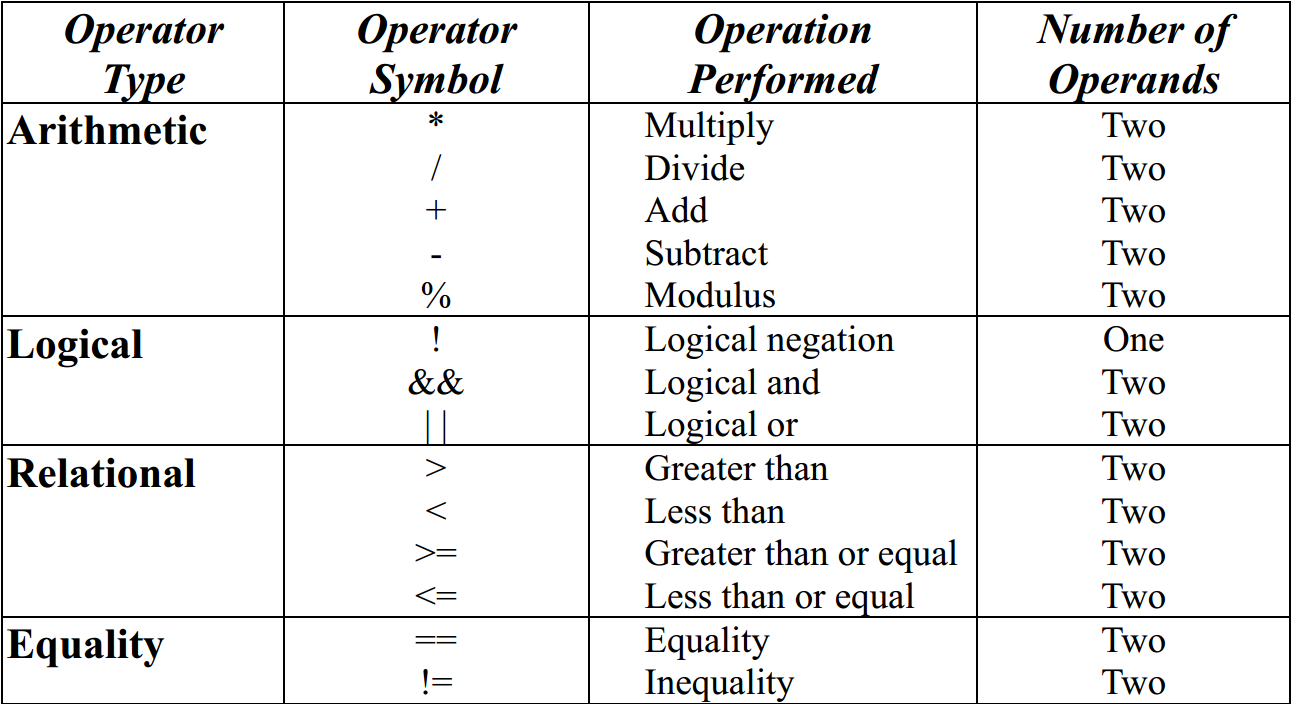# Trung tâm đào tạo thiết kế vi mạch Semicon

• Đăng ký
 Name: * Tên đăng nhập: * Email: * Mật khẩu: * Verify Password: * Fields marked with an asterisk (*) are required.## Verilog HDL Syntax And Semantics Part-IINumbers in Verilog

You can specify constant numbers in decimal, hexadecimal, octal, or binary format. Negative numbers are represented in 2's complement form.

When used in a number, the question mark (?) character is the Verilog alternative for the z character. The underscore character (_) is legal anywhere in a number except as the first character, where it is ignored.

 Integer Numbers . Verilog HDL allows integer numbers to be specified as .
• Sized or unsized numbers (Unsized size is 32 bits)
• Radix and hex digits (a,b,c,d,e,f) are case insensitive
• Spaces are allowed between the size, radix and value

Syntax: ';

Example of Integer Numbers

.

 Integer Stored as 1 00000000000000000000000000000001 8'hAA 10101010 6'b10_0011 100011 'hF 00000000000000000000000000001111

Verilog expands filling the specified by working from right-to-left

• When is smaller than , then leftmost bits of are truncated
• When is larger than , then leftmost bits are filled, based on the value of the leftmost bit in .
• Leftmost '0' or '1' are filled with '0'
• Leftmost 'Z' are filled with 'Z'
• Leftmost 'X' are filled with 'X'
 Note : X Stands for unknown and Z stands for high impedance, 1 for logic high or 1 and 0 for logic low or 0.

Example of Integer Numbers

 Integer Stored as 6'hCA 001010 6'hA 001010 16'bZ ZZZZZZZZZZZZZZZZ 8'bx xxxxxxxx

Real Numbers

• Verilog supports real constants and variables
• Verilog converts real numbers to integers by rounding
• Real Numbers can not contain 'Z' and 'X'
• Real numbers may be specified in either decimal or scientific notation
• .
• E
• Real numbers are rounded off to the nearest integer when assigning to an integer

Example of Real Numbers

 Real Number Decimal notation 1.2 1.2 0.6 0.6 3.5E6 3,500000.0

Signed and Unsigned Numbers
Verilog Supports both types of numbers, but with certain restrictions. Like in C language we don't have int and unint types to say if a number is signed integer or unsigned integer.

 Any number that does not have negative sign prefix is a positive number. Or indirect way would be "Unsigned". .

Negative numbers can be specified by putting a minus sign before the size for a constant number, thus they become signed numbers. Verilog internally represents negative numbers in 2's complement format. An optional signed specifier can be added for signed arithmetic

Examples

 Number Description 32'hDEAD_BEEF Unsigned or signed positive number -14'h1234 Signed negative number

The example file below shows how Verilog treats signed and unsigned numbers.

1 module signed_number;

2

3 reg [31:0]  a;

4

5 initial begin

6   a = 14'h1234;

7   \$display ("Current Value of a = %h", a);

8   a = -14'h1234;

9   \$display ("Current Value of a = %h", a);

11   \$display ("Current Value of a = %h", a);

13   \$display ("Current Value of a = %h", a);

14    #10  \$finish;

15 end

16

17 endmodule

Current Value of a = 00001234

Current Value of a = ffffedcc

Current Value of a = deadbeef

Current Value of a = 21524111

Bạn Có Đam Mê Với Vi Mạch hay Nhúng      -     Bạn Muốn Trau Dồi Thêm Kĩ Năng

Mong Muốn Có Thêm Cơ Hội Trong Công Việc

Và Trở Thành Một Người Có Giá Trị Hơn

# Hãy Để Chúng Tôi Hỗ Trợ Cho Bạn. SEMICONLần cập nhật cuối ( Thứ bảy, 11 Tháng 9 2021 13:55 )